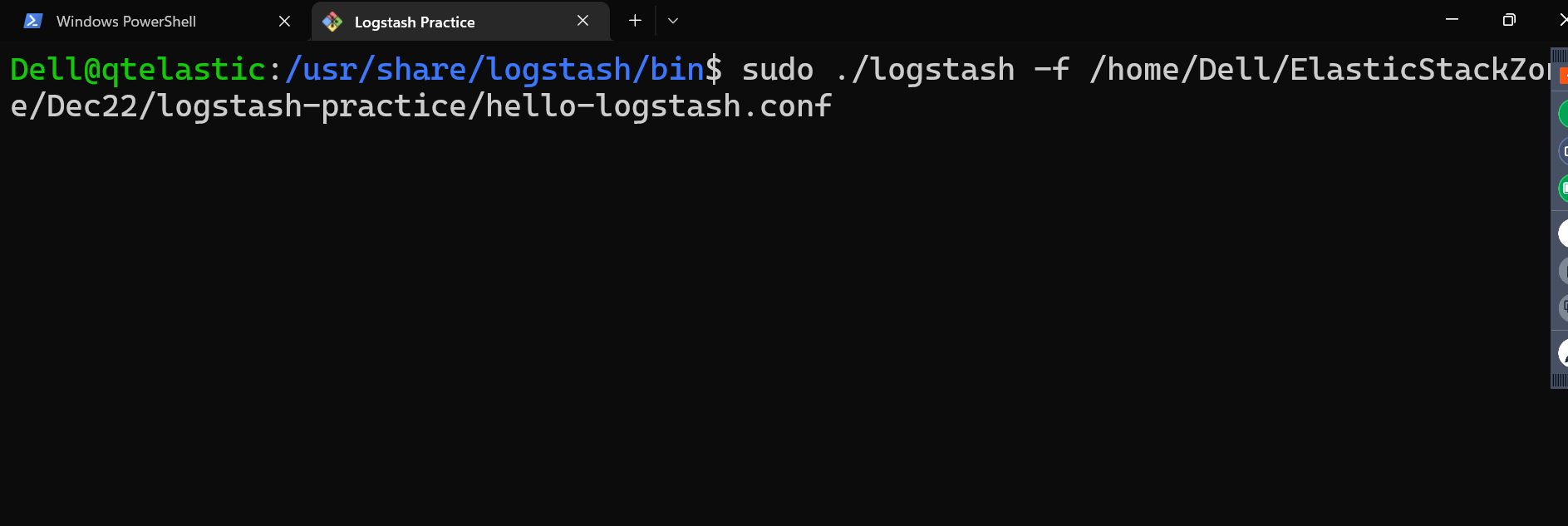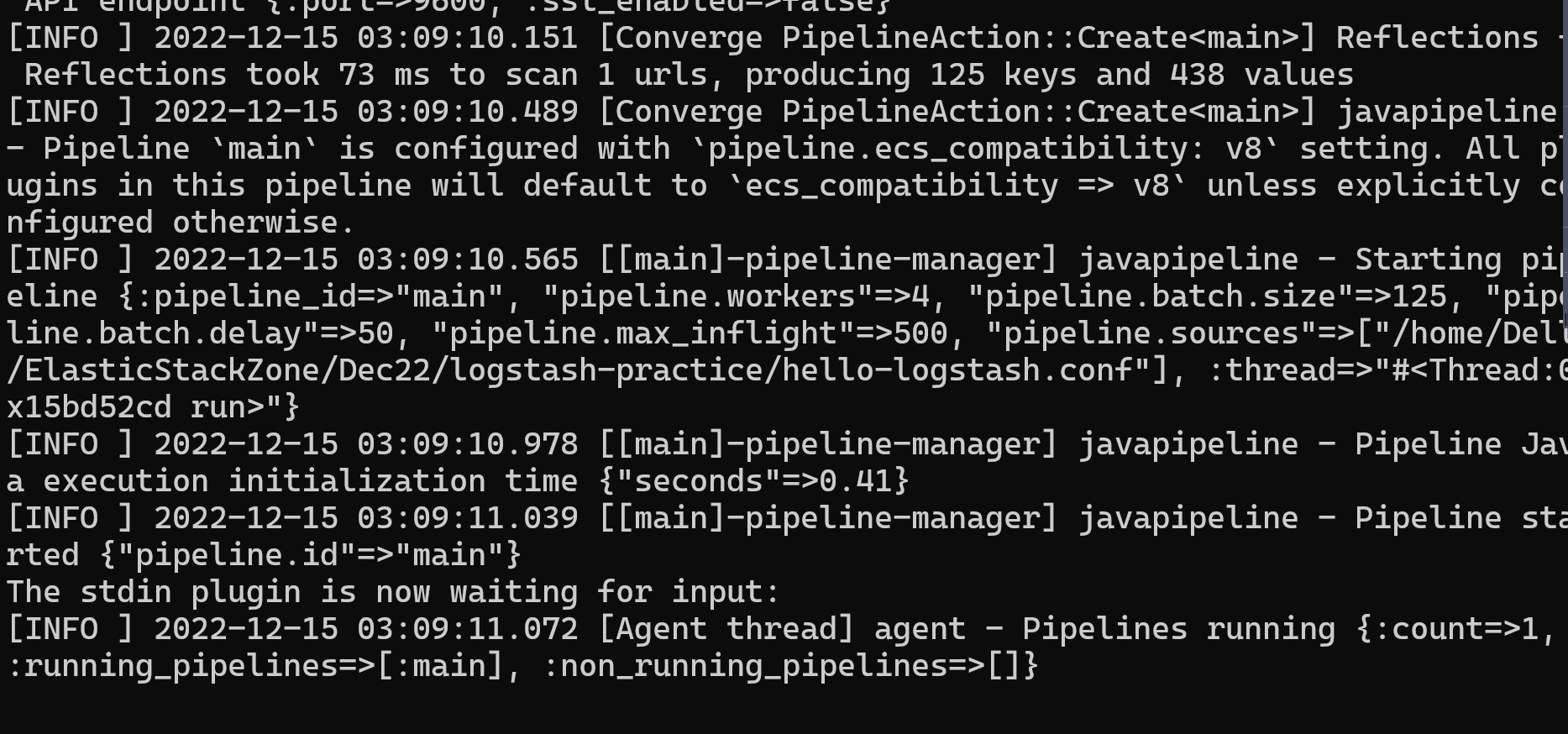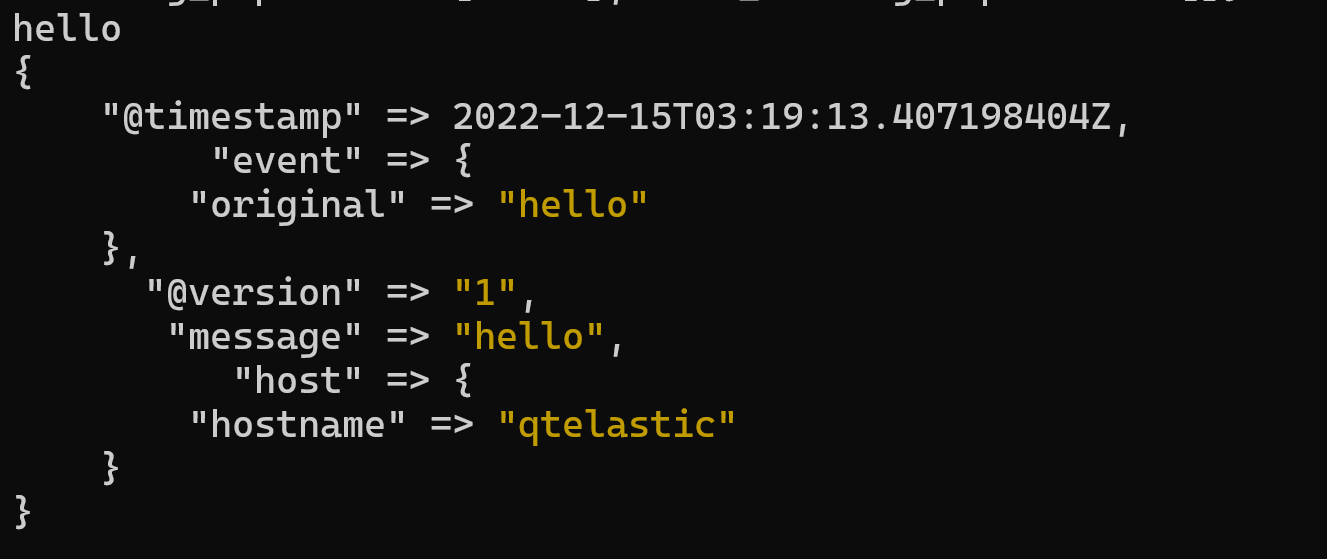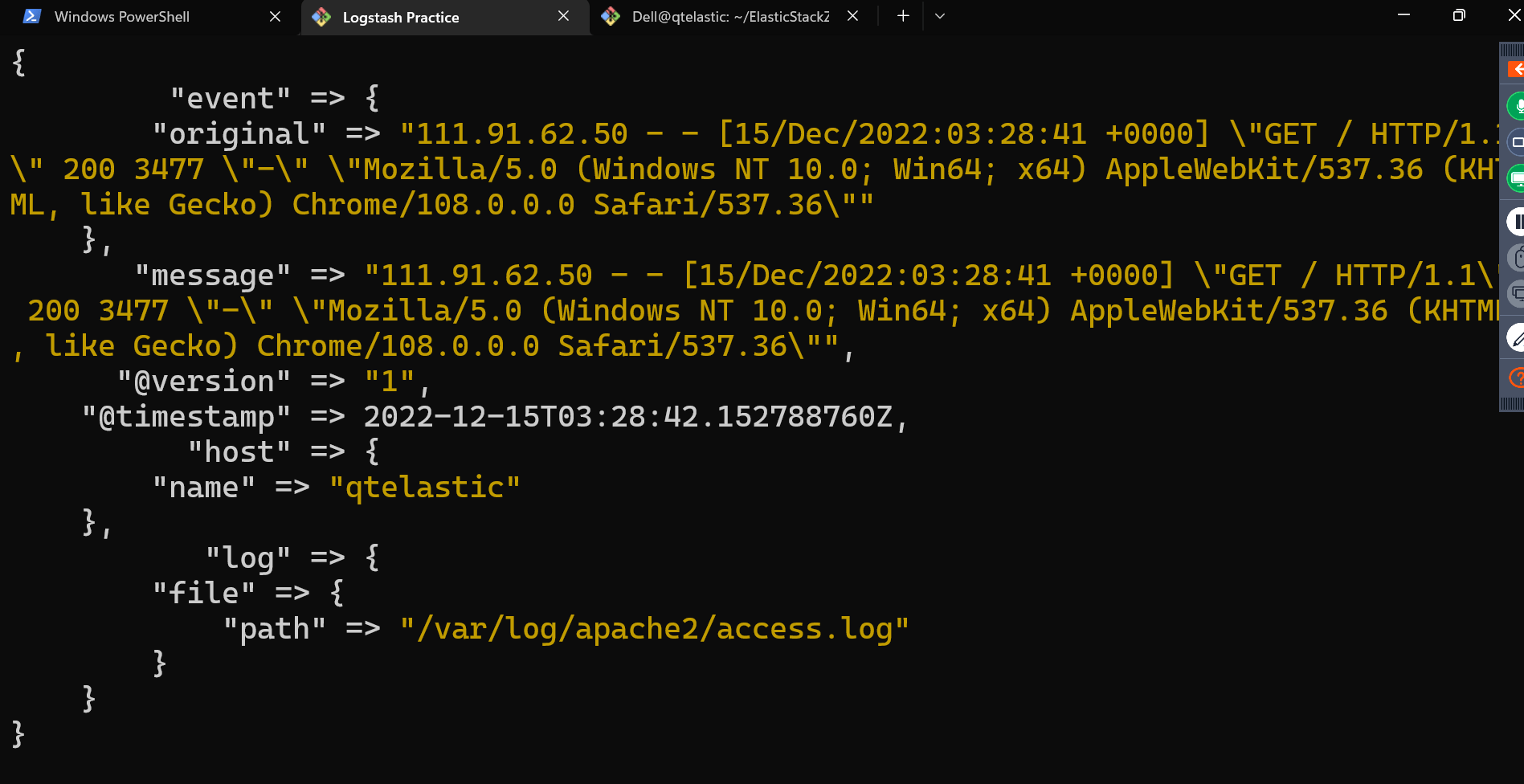## Log Parsing using logstash

• Lets install logstash Refer Here
• Logstash creates a pipeline based on conf file. The structure of conf file
``````input
{
<input-plugin-1> {
<parameter-1> => <value-1>
..
..
<parameter-n> => <value-n>
}
<input-plugin-n> {
<parameter-1> => <value-1>
..
..
<parameter-n> => <value-n>
}
}
output {
<output-plugin-1> {
<parameter-1> => <value-1>
..
..
<parameter-n> => <value-n>
}
<output-plugin-n> {
<parameter-1> => <value-1>
..
..
<parameter-n> => <value-n>
}

}
``````
• The input and output sections are mandatory. Filter section is optional
``````input
{
<input-plugin-1> {
<parameter-1> => <value-1>
..
..
<parameter-n> => <value-n>
}
<input-plugin-n> {
<parameter-1> => <value-1>
..
..
<parameter-n> => <value-n>
}
}

filter {
<filter-plugin-1> {
<parameter-1> => <value-1>
..
..
<parameter-n> => <value-n>
}
<filter-plugin-n> {
<parameter-1> => <value-1>
..
..
<parameter-n> => <value-n>
}

}
output {
<output-plugin-1> {
<parameter-1> => <value-1>
..
..
<parameter-n> => <value-n>
}
<output-plugin-n> {
<parameter-1> => <value-1>
..
..
<parameter-n> => <value-n>
}

}
``````

## Example-1: Lets try writing a very simple configuration to read from standard input (STDIN) and write to standard output (STDOUT)

• Refer Here for the conf file
• logstash binaries/executables are present in `/usr/share/logstash` and the logstash binary is present `/usr/share/logstash/bin`
• Refer Here for running logstash from command line
• Now the command would be
``````sudo /usr/share/logstash/bin/logstash -f hello-logstash.conf
``````* This configuration file is helpful in checking the logstash’s basic functionality
* Lets understand codecs in logstash Refer Here
* Refer Here for the two codecs used.
* Run ruby debug* Run json* Lets read the access logs of apache server Refer Here for the conf file* Exercise: Try to also write logs to /tmp/test.log
* Lets try to do a basic filter Refer Here

This site uses Akismet to reduce spam. Learn how your comment data is processed.